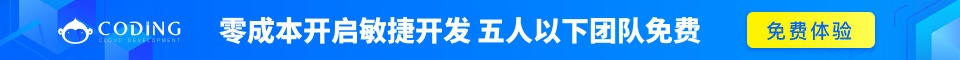# PHP While 循环

## PHP 循环

• while - 只要指定的条件成立，则循环执行代码块
• do...while - 首先执行一次代码块，然后在指定的条件成立时重复这个循环
• for - 循环执行代码块指定的次数
• foreach - 根据数组中每个元素来循环代码块

## while 循环

while 循环将重复执行代码块，直到指定的条件不成立。

while (条件)
{

}

### 实例

<html>
<body>

<?php
\$i=1;
while(\$i<=5)
{
echo "The number is " . \$i . "<br>";
\$i++;
}
?>

</body>
</html>

The number is 1
The number is 2
The number is 3
The number is 4
The number is 5

## do...while 语句

do...while 语句会至少执行一次代码，然后检查条件，只要条件成立，就会重复进行循环。

do
{

}
while (条件);

### 实例

<html>
<body>

<?php
\$i=1;
do
{
\$i++;
echo "The number is " . \$i . "<br>";
}
while (\$i<=5);
?>

</body>
</html>

The number is 2
The number is 3
The number is 4
The number is 5
The number is 6

for 循环和 foreach 循环将在下一章进行讲解。